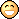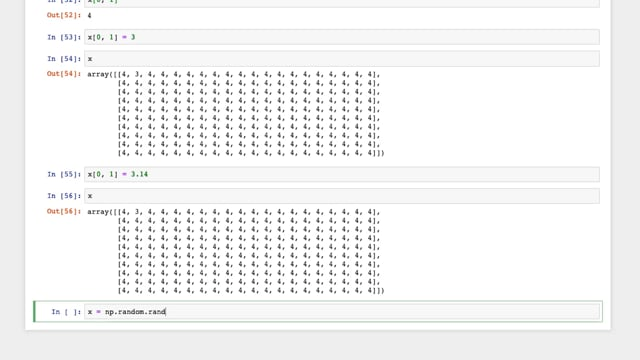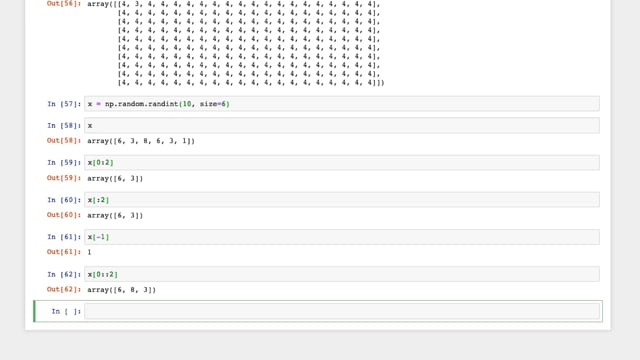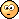Mis à jour le 04/10/2021

## Operate on NumPy arrays

Connectez-vous ou inscrivez-vous gratuitement pour bénéficier de toutes les fonctionnalités de ce cours !Hello and welcome to this course!Now that you have a solid grasp of Python Basics, let's dig a little deeper into its specific functionalities. By now you know that Python is the language of choice among many data scientists.

But what makes it stand out from the rest of the pack?

Well, that's to do with its ecosystem, that is, a combination of its `tools` , its `community` , and its `libraries` .

In fact, Python has libraries for almost every conceivable task, including:

• Numpy and SciPy for numerical calculations

• Matplotlib and Seaborn for plotting

• Scikit-learn for machine learning algorithms

• Pandas for dealing with large datasets (load, applying relational algebra operations, etc.)

• TensorFlow and PyTorch for deep learning

•  Etc.

In this part of the course, we're going to learn how to operate on Numpy arrays, create graphs with Matplotlib, and visually explore data using Seaborn.

As a starting point, in this chapter, I will give you an overview of the techniques used to efficiently load, store and manipulate data with NumPy! Ready? Then, let's go!

Why is it a good idea to use NumPy rather than basic Python data structures and functions?

Data can come from many different sources, but often we can consider them as arrays (or grids) of numbers. For instance, an image can be seen as a two dimensional array (or matrix) where each cell represents the intensity of a pixel. Being able to efficiently handle these arrays is really important, and NumPy is what allows us to do so.

NumPy stands for Numerical Python and provides us with an interface for operating on numbers. From a user point of view, NumPy arrays behave similarly to Python lists. However, it is much faster to operate on NumPy arrays, especially when they are large. NumPy arrays are at the foundation of the whole Python data science ecosystem.

Let's start by importing NumPy:

``````import numpy as np
``````

Unlike Python lists, NumPy arrays can only hold one specific type of data. The exact type of array is automatically worked out at its creation, and has an impact on the operations that can be performed on it. You can also specify the type manually. We'll see examples of each shortly.

You can create NumPy arrays in several ways:

#### ...From a Python list

``````# Array of integers:
np.array([1, 4, 2, 5, 3])
``````

If the original list holds different types of data, NumPy will try to convert everything to the most general type. For instance, integers (  `int`  ) may be converted to floating point numbers (`float`).

``````np.array([3.14, 4, 2, 3])
``````
`array([ 3.14, 4. , 2. , 3. ])`

And if you want to manually set the data type:

``````np.array([1, 2, 3, 4], dtype='float32')
``````

Unlike Python lists, NumPy arrays can be explicitly multidimensional. This means that NumPy recognizes multidimensional tables (for example, a table of numbers with rows and columns).

However, in native Python we represent a multidimensional array with a list of lists because, simply put, a table with 2 entries (rows and columns), is nothing more than a list of rows, and a row is a list of numbers!

``````# A list of lists in converted to a 2-dimensional array
original_list = [[1,2,3],[3,4,5],[6,7,8]]
two_dimensional_array = np.array(original_list)
``````

#### ...Manually

It's often more efficient, especially for large arrays, to create them yourself. NumPy provides us with quite a few ways to do this:

``````# An array of length 10, filled with 0:
np.zeros(10, dtype=int)
# An array of size 3x5 filled with 1.0 (float)
np.ones((3, 5), dtype=float)
# An array of size 3x5 filled with 3.14
np.full((3, 5), 3.14)
# An array containing a linear sequence starting at 0 and
# going up to 20, with steps of 2
np.arange(0, 20, 2)
# An array of 5 numbers, linearly spaced between 0 and 1
np.linspace(0, 1, 5)
# An array of the given shape and populate it with random
# samples. You can also try using "randint" and "normal"
np.random.random((3, 3))
# The identity matrix of size 3
np.eye(3)
``````

NumPy arrays have some very useful properties! For example, if you want to know how many dimensions an array has, you can use  `.ndim` . To confirm the dimension of a shape, you can also look at its shape, using  `.shape` . To look at the size of the array, or in other words, to see how many elements it has, we can use  `.size`. Finally, if we want to see the data type of the elements, we can look at that using  `.dtype`

``````np.random.seed(0)
x1 = np.random.randint(10, size=6)
# 1-dimensional array
print("Number of dimensions: ", x1.ndim)
print("Shape: ", x1.shape)
print("Size: ", x1.size)
print("Type: ", x1.dtype)
``````#### Accessing a single element

You'll often need to access specific elements of an array. This is called indexing. Fortunately, this is really easy with NumPy!

``````print(x1)
# The first element
print(x1)
# The last element
print(x1[-1])

x2 = np.random.randint(10, size=(3, 4))
# 2-dimensional array
print(x2[0,1])

# You can also modify values:
x1 = "1000"
print(x1)

# Mind the type
x1 = 3.14
print(x1)
``````

#### Accessing several elements

To extract a contiguous subset of a string, you can combine `[]`  and `:` to access several elements at once. This is called slicing. The syntax is simple :  `x[begin:end:step]`

``````# First 5 elements
print(x1[:5])
# Elements from the 6th on
print(x1[5:])
# Every two elements
print(x1[::2])
``````
``````x1[::-1]
``````

You can also access elements of a multidimensional array. For instance, to access the first row of a matrix:

``````print(x2)
x2[0,:]
``````

#### Concatenating arrays

You can concatenate (or join) two or more arrays:

``````x = np.array([1, 2, 3])
y = np.array([3, 2, 1])
np.concatenate([x, y])
``````

If the arrays are multidimensional, you can use either `vstack`  (vertical) or  `hstack`  (horizontal).

``````x = np.array([1, 2, 3])
grid = np.array([[9, 8, 7], [6, 5, 4]])
np.vstack([x, grid])
``````So far in this chapter, we have explored basics of using NumPy arrays. Now, we'll be looking at what makes NumPy essential.

CPython, the reference implementation of Python, is very flexible. However, this flexibility prevents it from using all possible optimizations. Check the execution time of this piece of code:

``````def calculate_inverse(values):
output = np.empty(len(values))
for i in range(len(values)):
output[i] = 1.0 / values[i]
return output

values = np.random.randint(1, 10, size=5)
print(calculate_inverse(values))

large_array = np.random.randint(1, 100, size=1000000)

# This is a Jupyter notebook tool to measure the execution
# time of an instruction
%timeit calculate_inverse(large_array)
``````

As you can see, it takes several seconds to complete a million operations. As today's processors can perform billions of operations per second, this amount of time seems absurd.This delay is due to all the additional operations the interpreter must perform, such as function calls and type checks.

In many cases, NumPy provides an interface for these operations that involves only the same type of data. This interface uses a C language implementation, which can access all the features of modern processors. For example, we can calculate the inverses of all the elements of a NumPy table like this:

``````%timeit (1.0 / large_array)
``````

This took about 1000 times less time on my machine.

But, what are these functions?

#### Universal functions

``````# Simple mathematics first
x = np.arange(4)
print("x     =", x)
print("x + 5 =", x + 5)
print("x - 5 =", x - 5)
print("x * 2 =", x * 2)
print("x / 2 =", x / 2)
# Divide and round
print("x // 2 =", x // 2)
``````

To apply functions on NumPy arrays :

``````x = [-2, -1, 1, 2]

print("Absolute value: ", np.abs(x))
print("Exponential: ", np.exp(x))
print("Logarithm: ", np.log(np.abs(x)))
``````

#### Boolean operations

You can also perform Boolean operations on your arrays. In other words, you can ask if a certain condition is true for each element of an array. For example:

``````x = np.random.rand(3,3)
x > 0.5
``````

You can couple this capability with  `np.where`, to return, among all the elements of an array, the indexes of those which have a certain property:

``````np.where(x > 0.5)
``````

#### Aggregation

Often, when dealing with large amounts of data, the first thing to do is to calculate statistics on these data, such as the mean or standard deviation. NumPy has functions for this.

``````L = np.random.random(100)
np.sum(L)
``````

This function also exists in Python, but the NumPy version is much faster. Similarly, NumPy has equivalents for  `min`  and  `max` . However, be careful with the optional arguments of each version of these functions. Also, keep in mind that only NumPy correctly handles multidimensional arrays.

``````%timeit sum(large_array)
%timeit np.sum(large_array)
``````

Aggregation functions can also be applied to only one dimension of a multidimensional array. For example, we may need the sum of the elements of each column in a matrix. To do this we use the optional `axis` argument.

``````M = np.random.random((3, 4))
print(M)
# Note the syntax variable.function instead of
# np.fonction(variable). Both are acceptable if
# variable is a Numpy array.
print("Sum of all elements of M: ", M.sum())
print("Sums of the columns of M: ", M.sum(axis=0))
``````

Among the many available functions, note:
-  `np.std`  For the standard deviation
-  `np.argmin`  For the index of the smallest element
-  `np.percentile`  To get statistics on the elements

Broadcasting refers to a set of rules for applying a transaction (that normally applies to only one value) to all members of a NumPy table. For example, for tables of the same size, operations such as addition normally apply element by element.

``````a = np.array([0, 1, 2])
b = np.array([5, 5, 5])
a + b
``````

Broadcasting allows us to apply these operations to tables of different sizes. For example:

``````a + 5
``````

It's as if NumPy had converted the value  `5`  into a table of size 3, and then added this table to the one contained in  `a`  . This is just a view designed to help you understand what's going on. NumPy does the addition without all these additional operations, which makes everything faster.

It also works when both arguments are tables:

``````M = np.ones((3, 3))
print("M is: \n", M)
print("M+a is: \n", M+a)
``````

Feel free to try these operations with tables of different sizes and dimensions to get the hang of this tool.

• You can create NumPy arrays from a Python List or manually.

• Indexing is used to access specific elements of an array.

• Slicing is used to extract a specific subset of a string.

• NumPy functions include:

• Universal functions

• Boolean operations

• Aggregation